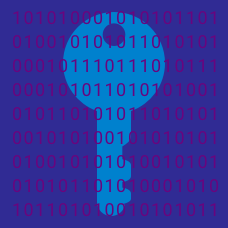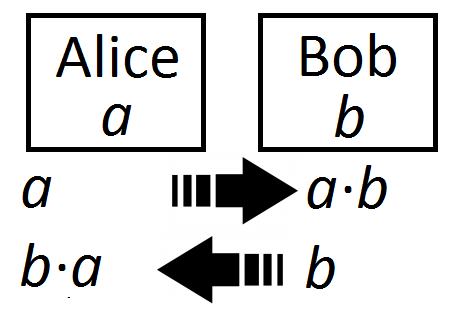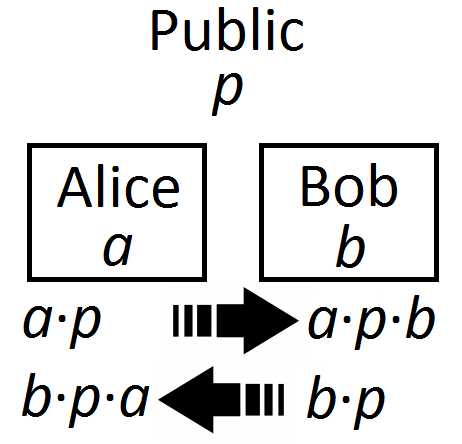Number Theory

# Public-Key EncryptionWhat sort of mathematical breakthrough would endanger the security of the RSA encryption algorithm?

In an encoding scheme, each letter is replaced as follows: $A = 1, \ B = 2, \ C = 3,\ \ldots\ , \ Z = 26.$ For example, $$CODE$$ would be encoded as $$31545,$$ since $$C = 3,$$ $$O = 15,$$ $$D = 4,$$ and $$E = 5.$$

What is the main problem with such a system?

To crack a code, Alice and Bob need to find the product of their secret large primes, $$a$$ and $$b,$$ so they each send these primes to each other.Alice receives $$b$$ and performs $$b \cdot a$$, while Bob receives $$a$$ and performs $$a \cdot b$$.

What's the main issue with this encryption system?

Suppose Alice and Bob want to establish an encryption key. They start by choosing a large prime $$p$$, which is shared in public.Alice secretly picks a large prime $$a$$, and Bob secretly picks a large prime $$b$$. Then both Alice and Bob multiply their primes by $$p$$, so Alice now has $$a \cdot p$$ and Bob has $$b \cdot p$$.

They send $$a \cdot p$$ and $$b \cdot p$$ to each other. They then multiply what they receive by what their own secret prime. That is, Alice receives $$b \cdot p$$ and performs $$b \cdot p \cdot a$$, and Bob receives $$a \cdot p$$ and performs $$a \cdot p \cdot b$$.

Alice and Bob now have the same number that they can use as their secret key.

Is this encryption system secure?

Suppose a cryptography system included the step where, given $$m ,$$ $$e ,$$ and $$n,$$ it is necessary to calculate $$c$$ in $$m^e \equiv c \pmod{n}.$$ (That is, first $$m^e$$ is found, then the value is divided by $$n$$ with the remainder $$c$$. )

$$e,$$ $$n,$$ and $$c$$ are going to be shared in public, and it is important that $$m$$ remains a secret.

Which of these circumstances (if known by someone trying to break the code) would easily compromise $$m?$$

×